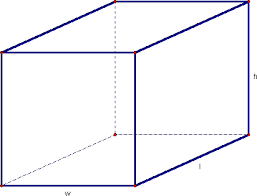## How to Calculate and Solve for the Width, Height, Length and Volume of a Rectangular Prism | Nickzom CalculatorThe image above is a rectangular prism.

To compute the volume of a rectangular prism requires three essential parameters which are the length, width and height of the rectangular prism.

V = lwh

Where;
V = Volume of the rectangular prism
l = Length of the rectangular prism
w = Width of the rectangular prism
h = Height of the rectangular prism

Let’s solve an example;
Find the volume of a rectangular prism with a length of 18 cm, a width of 12 cm and a height of 21 cm.

This implies that;
l = Length of the rectangular prism = 18 cm
w = Width of the rectangular prism = 12 cm
h = Height of the rectangular prism = 21 cm

V = lwh
V = 18 x 12 x 21
V = 4536

Therefore, the volume of the rectangular prism is 4536 cm3.

Calculating the Length of a Rectangular Prism using the Volume, Width and Height of the Rectangular Prism.

l = V / wh

Where;
l = Length of the rectangular prism
V = Volume of the rectangular prism
w = Width of the rectangular prism
h = Height of the rectangular prism

Let’s solve an example;
Find the length of a rectangular prism with a width of 19 cm, a height of 24 cm and a Volume of 250 cm3.

This implies that;
w = Width of the rectangular prism = 19 cm
h = Height of the rectangular prism = 24 cm
V = Volume of the rectangular prism = 250 cm3

l = V / wh
l = 250 / 19 x 24
l = 250 / 456
l = 0.55

Therefore, the length of the rectangular prism is 0.55 cm.